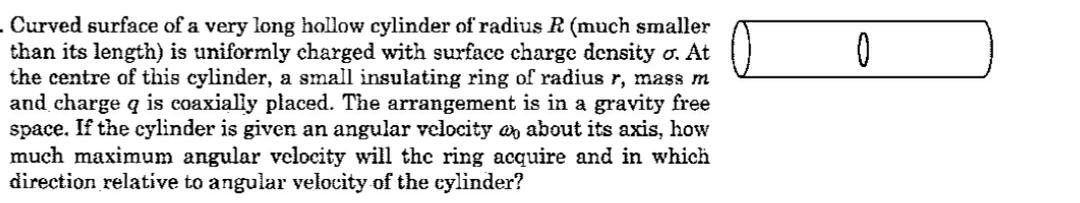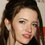# An interesting EMI problem

Here is a problem :Here is the solution : First we will calculate the magnetic field at any random point inside the cylinder due to rotation. Because rotating charges creates current
We will take a elemental ring of width $dx$ by moving $x$ $dA=2πRdx$ $dq=\sigma 2πRdx$ $I=\frac{Q \omega }{2π}$
$\boxed{I=\mu_{0} \sigma R \omega }$ $\int dB=\int_{- \infty}^{+\infty} \frac{\mu_{0} \sigma R^{3} \omega dx}{2(x^{2}+R^{2})^{1.5}}$
After integration $B= \mu_{0} \sigma R \omega$
This is basically a induced magnetic field due to rotating of charges if cylinder .
now due to this rotation, there will an electric field induced $E= \frac{r}{2} \frac{dB}{dt}$ $E=\frac{a}{2} \frac{\mu_{0} \sigma R \omega }{dt }$ Now due to acting of electric field in ring, there will act a torque in ring $\tau=qEa$ $\tau=qa \frac{a \mu_{0} \sigma R \omega }{2dt}$ $\tau dt=qa \frac{a \mu_{0} \sigma R \omega }{2}$
Let , due to this torque,there will be an instant maximum angular velocity $\omega_{0}$ in the ring $\tau dt =I_{ring} \omega_{0}$ $I_{ring} \omega _{0} =qa \frac{a \mu_{0} \sigma R \omega }{2}$
Now from here maximum angular velocity $\omega _{0}$ comes as $\boxed{\omega_{0}=\frac{\mu_{0}q \sigma R \omega }{2m}}$

Feel free to ask anything regarding the solution and problem.

If you have another trick/method to solve this particular problem please share it in below coments.Note by Talulah Riley
4 months ago

This discussion board is a place to discuss our Daily Challenges and the math and science related to those challenges. Explanations are more than just a solution — they should explain the steps and thinking strategies that you used to obtain the solution. Comments should further the discussion of math and science.

When posting on Brilliant:

• Use the emojis to react to an explanation, whether you're congratulating a job well done , or just really confused .
• Ask specific questions about the challenge or the steps in somebody's explanation. Well-posed questions can add a lot to the discussion, but posting "I don't understand!" doesn't help anyone.
• Try to contribute something new to the discussion, whether it is an extension, generalization or other idea related to the challenge.

MarkdownAppears as
*italics* or _italics_ italics
**bold** or __bold__ bold
- bulleted- list
• bulleted
• list
1. numbered2. list
1. numbered
2. list
Note: you must add a full line of space before and after lists for them to show up correctly
paragraph 1paragraph 2

paragraph 1

paragraph 2

[example link](https://brilliant.org)example link
> This is a quote
This is a quote
    # I indented these lines
# 4 spaces, and now they show
# up as a code block.

print "hello world"
# I indented these lines
# 4 spaces, and now they show
# up as a code block.

print "hello world"
MathAppears as
Remember to wrap math in $$ ... $$ or $ ... $ to ensure proper formatting.
2 \times 3 $2 \times 3$
2^{34} $2^{34}$
a_{i-1} $a_{i-1}$
\frac{2}{3} $\frac{2}{3}$
\sqrt{2} $\sqrt{2}$
\sum_{i=1}^3 $\sum_{i=1}^3$
\sin \theta $\sin \theta$
\boxed{123} $\boxed{123}$

Sort by: